#### Two-Way Analysis of Variance

Two-Way ANOVA:

Two-way analysis of variance is the extension to one-way analysis of variance. There are two independent variables (therefore the name two-way).

Assumptions:

a) The populations from which the samples were obtained should be normally or about normally distributed.
b) The samples should be independent.
c) The variances of populations should be equivalent.
d) The groups should have similar sample size.

Hypotheses:

In a two-way ANOVA, there are three sets.

The null hypotheses for each and every set are shown below:

a) The population means of first factor are equivalent. This is similar to the one-way ANOVA for row factor.
b) The population means of second factor are equivalent. This is similar to the one-way ANOVA for the column factor.
c) There is no interaction among the two factors. This is identical to executing a test for independence with the contingency tables.

Factors:

In a two-way ANOVA, the two independent variables are termed as factors. The idea is that, there are two variables or factors, which influence the dependent variable. Each and every factor will encompass two or more levels in it, and the degree of freedom for all factors is one less than the number of levels.

Treatment Groups:

Treatement Groups are made by making all possible combinations of the two factors. For illustration, when the first factor consists of 3 levels and the second factor consists of 2 levels, and then there will be 3 x 2 = 6 different treatment groups.

As an illustration, let's suppose we are planting corn. The kind of seed and kind of fertilizer are the two factors we are considering in this illustration. This illustration consists of 15 treatment groups. There are 3 – 1 = 2 degree of freedom for the kind of seed, and 5 – 1 = 4 degree of freedom for the kind of fertilizer. There are 2*4 = 8 degree of freedom for the interaction between the kind of seed and kind of fertilizer.

The data which really appears in the table are samples. In this condition, 2 samples from each and every treatment group were taken.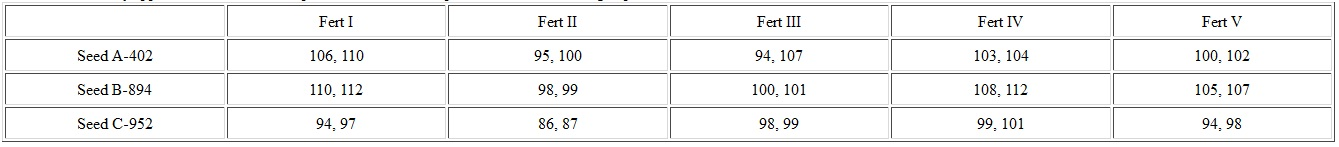Main Effect:

The main effect includes the independent variables one at a time. The interaction is ignored for this portion. Just the rows columns are utilized, not mixed. This is the portion that is identical to the one-way analysis of variance. Each of the variances computed to analyze the main effects are similar to the between variances.

Interaction Effect:

This can be defined as the effect which one factor has on the other factor. The degree of freedom here is the product of two degrees of freedom for each and every factor.

Within Variation:

This variation is the sum of squares in each and every treatment group. We have one less than the sample size (recall all the treatment groups should have similar sample size for a two-way ANOVA) for each and every treatment group. Total number of treatment groups is the product of number of levels for each and every factor. Within variance is the within variation divided by its degree of freedom.

The within group is as well termed as the error.

F-Tests:

There is an F-test for each and every hypothesis and the F-test is the mean square for each of the main effect and the interaction effect divided by within variance. The numerator degree of freedom come from each effect and the denominator degree of freedom is the degree of freedom for within variance in each and every case.

Two-Way ANOVA Table:

It is supposed that the main effect A consists of a levels (and A = a - 1 df), main effect B consists of b levels (and B = b - 1 df), n is the sample size of each and every treatment and N = abn is the net sample size. Note that the overall degree of freedom is once again one less than the net sample size.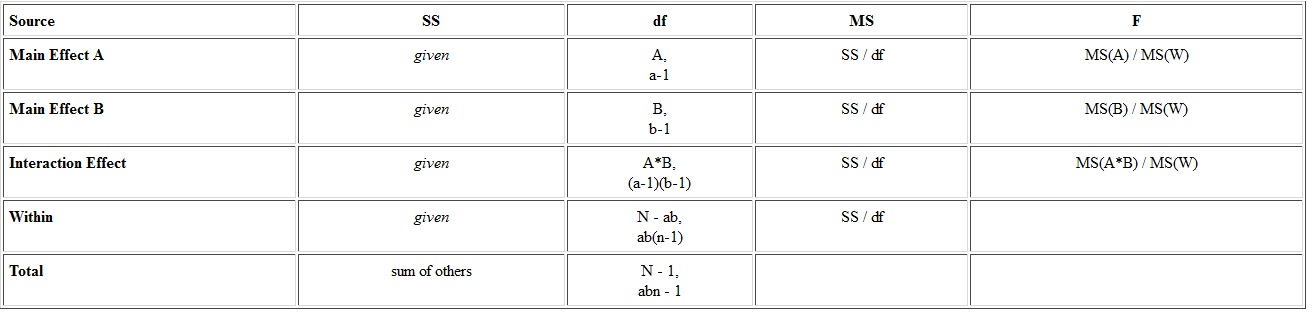Summary:

The given outcomes are computed by using the Quattro Pro spread-sheet. It gives the p-value and critical values are for alpha = 0.05.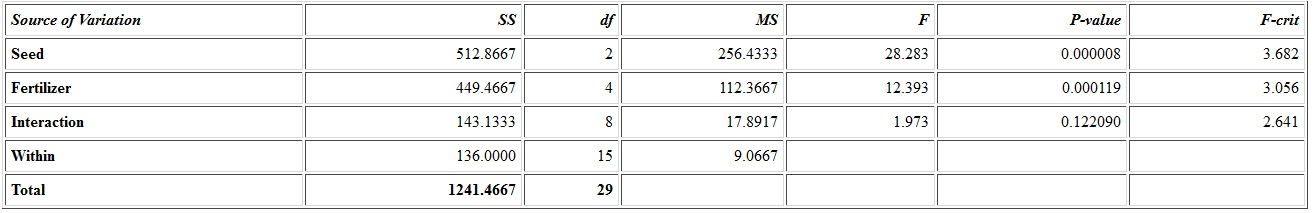From the above outcomes, we can observe that the main effects are both significant; however the interaction between them is not. That is, the kinds of seed are not all equivalent, and the kinds of fertilizer are not all equal, however the kind of seed does not interact with the kind of fertilizer.

Error in Bluman Textbook:

The two-way ANOVA, in the Bluman text consists of the wrong values in it. The student would encompass no way of knowing this as the book does not elucidate how to compute the values.

Here is the accurate table: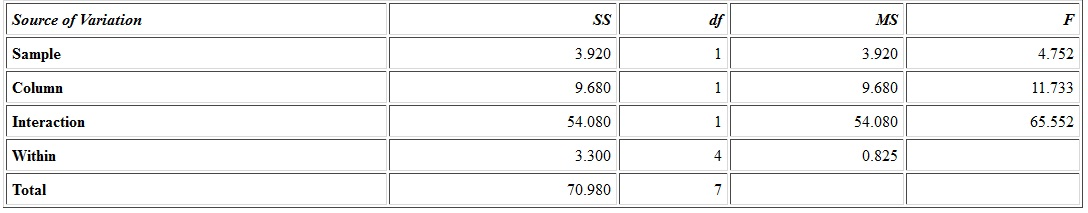The student will be accountable for finishing the table, not for coming-up with the sum of squares that go to the table in the first position.

Central Limit Theorem:

Sampling Distribution of the Sample Means:

Rather than working with individual scores, the statisticians frequently work with means. What happens is that some samples are taken, the mean is calculated for each and every sample, and then the means are employed as the data, instead of individual scores being employed. The sample is a sampling distribution of sample means.

Whenever all of the possible sample means are calculated, then the given properties are true:

a) The mean of sample means will be the mean of population.
b) The variance of sample means will be the variance of population divided by the sample size.
c) The standard deviation of sample means (termed as the standard error of mean) will be smaller than the population mean and equivalent to the standard deviation of population divided by the square root of sample size.

When the population consists of a normal distribution, then the sample means will encompass a normal distribution.

When the population is not normally distributed, however the sample size is adequately big, then the sample means will encompass an approximately normal distribution. Some of the books define adequately big as at least 30 and others as at least 31.

The formula for z-score whenever working with the sample means is as follows: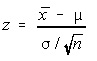Finite Population Correction Factor:

When the sample size is greater than 5% of the population size and the sampling is completed with no replacement, then a correction requires to be made to the standard error of means.
In the given formula, N is the population size and n is sample size. The adjustment is to multiply the standard error by square root of the quotient of difference between the population and sample sizes and one less than population size.

For most of the part, we will be avoiding this.

Latest technology based Statistics Online Tutoring Assistance

Tutors, at the www.tutorsglobe.com, take pledge to provide full satisfaction and assurance in Statistics help via online tutoring. Students are getting 100% satisfaction by online tutors across the globe. Here you can get homework help for Statistics, project ideas and tutorials. We provide email based Statistics help. You can join us to ask queries 24x7 with live, experienced and qualified online tutors specialized in Statistics. Through Online Tutoring, you would be able to complete your homework or assignments at your home. Tutors at the TutorsGlobe are committed to provide the best quality online tutoring assistance for Statistics Homework help and assignment help services. They use their experience, as they have solved thousands of the Statistics assignments, which may help you to solve your complex issues of Statistics. TutorsGlobe assure for the best quality compliance to your homework. Compromise with quality is not in our dictionary. If we feel that we are not able to provide the homework help as per the deadline or given instruction by the student, we refund the money of the student without any delay.